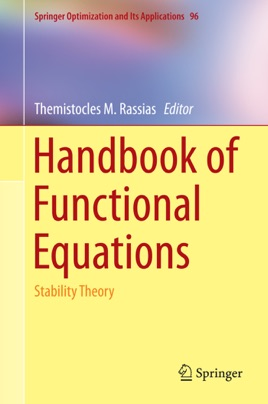• \$99.99

## Publisher Description

This  handbook consists of seventeen chapters written by eminent scientists from the international mathematical community, who present important research works in the field of mathematical analysis and related subjects, particularly in the Ulam stability theory of functional equations. The book provides an insight into a large domain of research with emphasis to the discussion of several theories, methods and problems in approximation theory, analytic inequalities, functional analysis, computational algebra and applications.                           The notion of stability of functional equations has its origins with S. M. Ulam, who posed the fundamental problem for approximate homomorphisms in 1940 and with D. H. Hyers, Th. M. Rassias, who provided the first significant solutions for additive and linear mappings in 1941 and 1978, respectively. During the last decade the notion of stability of functional equations has evolved into a very active domain of mathematical research with several applications of interdisciplinary nature.                                                                                         The chapters of this handbook focus mainly on both old and recent developments on the equation of homomorphism for square symmetric groupoids, the linear and polynomial functional equations in a single variable, the Drygas functional equation on amenable semigroups, monomial functional equation,  the Cauchy–Jensen type mappings, differential equations and differential operators, operational equations and inclusions, generalized module left higher derivations, selections of set-valued mappings, D’Alembert’s functional equation, characterizations of information measures,  functional equations in restricted domains, as well as generalized functional stability and fixed point theory.